## Angles related to Parallel Lines and Transversals

 8.3 Angles related to Parallel Lines and Transversals

Parallel lines:

 Definition Parallel lines will not intersect with each other. Parallel lines can be denoted using arrowheads as shown in the diagram below.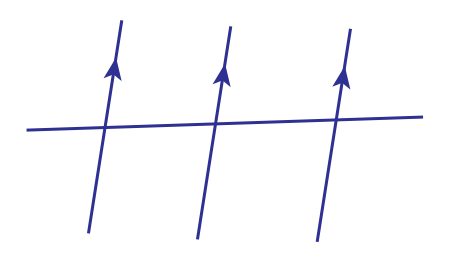Transversal:

 Definition A straight line that intersects two or more straight lines. The diagram below shows that $$PQ$$ is a transversal.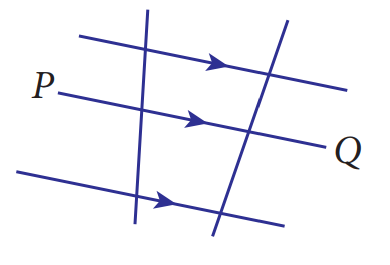The corresponding angles, alternate angles and interior angles:

(i) Corresponding angles

• The corresponding angles are equal where $$\angle a=\angle b$$.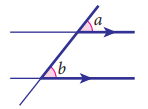(ii) Alternate angles

• The alternate angles are equal where $$\angle b=\angle c$$.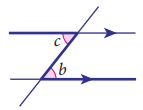(iii) Interior angles

• The sum of the interior angles is $$180^\circ$$ where $$\angle c+\angle d=180^\circ$$.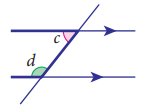Angles of elevation and depression:

(i) Angle of elevation

 Definition When a person sees an object which is at a higher level, the angle between the line of sight and the horizontal line is known as the angle of elevation.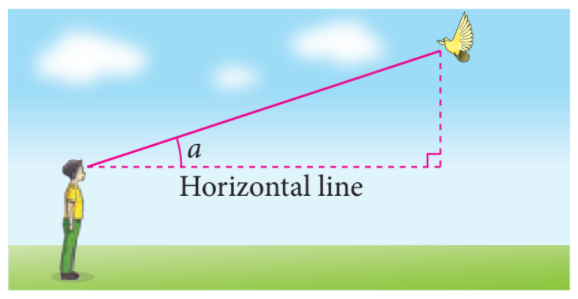Form the diagram above, angle $$a$$ is the angle of elevation of the bird from the sight of the boy.

(ii) Angle of Depression

 Definition When a person sees an object which is at a lower level, the angle between the line of sight and the horizontal line is known as the angle of depression.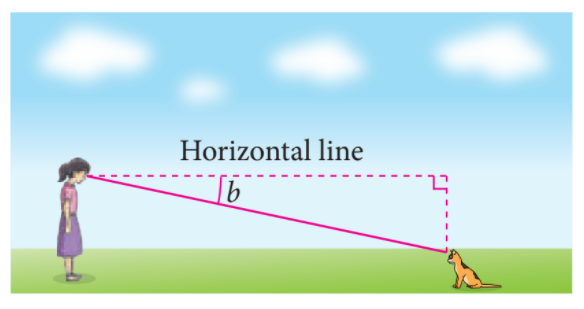Form the diagram above, angle $$b$$ is the angle of depression of the cat from the sight of the girl.